# Year 5 programmes of study

Number - number and place value

Pupils should be taught to:

• read, write, order and compare numbers to at least 1,000,000 and determine the value of each digit
• count forwards or backwards in steps of powers of 10 for any given number up to 1,000,000
• interpret negative numbers in context, count forwards and backwards with positive and negative whole numbers, including through 0
• round any number up to 1,000,000 to the nearest 10, 100, 1,000, 10,000 and 100,000
• solve number problems and practical problems that involve all of the above
• read Roman numerals to 1,000 (M) and recognise years written in Roman numerals

Pupils should be taught to:

• add and subtract whole numbers with more than 4 digits, including using formal written methods (columnar addition and subtraction)
• add and subtract numbers mentally with increasingly large numbers
• use rounding to check answers to calculations and determine, in the context of a problem, levels of accuracy
• solve addition and subtraction multi-step problems in contexts, deciding which operations and methods to use and why

Number - multiplication and division

Pupils should be taught to:

• identify multiples and factors, including finding all factor pairs of a number, and common factors of 2 numbers
• know and use the vocabulary of prime numbers, prime factors and composite (non-prime) numbers
• establish whether a number up to 100 is prime and recall prime numbers up to 19
• multiply numbers up to 4 digits by a one- or two-digit number using a formal written method, including long multiplication for two-digit numbers
• multiply and divide numbers mentally, drawing upon known facts
• divide numbers up to 4 digits by a one-digit number using the formal written method of short division and interpret remainders appropriately for the context
• multiply and divide whole numbers and those involving decimals by 10, 100 and 1,000
• recognise and use square numbers and cube numbers, and the notation for squared (²) and cubed (³)
• solve problems involving multiplication and division, including using their knowledge of factors and multiples, squares and cubes
• solve problems involving addition, subtraction, multiplication and division and a combination of these, including understanding the meaning of the equals sign
• solve problems involving multiplication and division, including scaling by simple fractions and problems involving simple rates

Number - fractions (including decimals and percentages)

Pupils should be taught to:

• compare and order fractions whose denominators are all multiples of the same number
• identify, name and write equivalent fractions of a given fraction, represented visually, including tenths and hundredths
• recognise mixed numbers and improper fractions and convert from one form to the other and write mathematical statements > 1 as a mixed number [for example,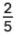+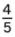=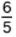= 1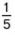]
• add and subtract fractions with the same denominator, and denominators that are multiples of the same number
• multiply proper fractions and mixed numbers by whole numbers, supported by materials and diagrams
• read and write decimal numbers as fractions [for example, 0.71 =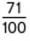]
• recognise and use thousandths and relate them to tenths, hundredths and decimal equivalents
• round decimals with 2 decimal places to the nearest whole number and to 1 decimal place
• read, write, order and compare numbers with up to 3 decimal places
• solve problems involving number up to 3 decimal places
• recognise the per cent symbol (%) and understand that per cent relates to ‘number of parts per 100’, and write percentages as a fraction with denominator 100, and as a decimal fraction
• solve problems which require knowing percentage and decimal equivalents of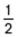,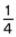,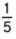,and those fractions with a denominator of a multiple of 10 or 25

Measurement

Pupils should be taught to:

• convert between different units of metric measure [for example, kilometre and metre; centimetre and metre; centimetre and millimetre; gram and kilogram; litre and millilitre]
• understand and use approximate equivalences between metric units and common imperial units such as inches, pounds and pints
• measure and calculate the perimeter of composite rectilinear shapes in centimetres and metres
• calculate and compare the area of rectangles (including squares), including using standard units, square centimetres (cm²) and square metres (m²), and estimate the area of irregular shapes
• estimate volume [for example, using 1 cm³ blocks to build cuboids (including cubes)] and capacity [for example, using water]
• solve problems involving converting between units of time
• use all four operations to solve problems involving measure [for example, length, mass, volume, money] using decimal notation, including scaling

Geometry - properties of shapes

Pupils should be taught to:

• identify 3-D shapes, including cubes and other cuboids, from 2-D representations
• know angles are measured in degrees: estimate and compare acute, obtuse and reflex angles
• draw given angles, and measure them in degrees (°)
• identify:
• angles at a point and 1 whole turn (total 360°)
• angles at a point on a straight line and half a turn (total 180°)
• other multiples of 90°
• use the properties of rectangles to deduce related facts and find missing lengths and angles
• distinguish between regular and irregular polygons based on reasoning about equal sides and angles

Geometry - position and direction

Pupils should be taught to:

• identify, describe and represent the position of a shape following a reflection or translation, using the appropriate language, and know that the shape has not changed

Statistics

Pupils should be taught to:

• solve comparison, sum and difference problems using information presented in a line graph
• complete, read and interpret information in tables, including timetables